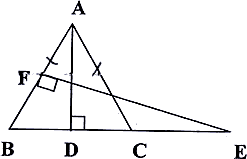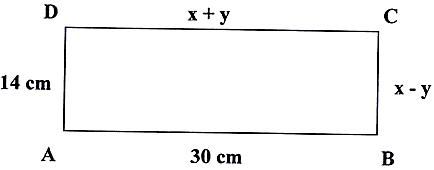Wednesday , October 5 2022# 10th Maths Periodic Test II (2018-19)

## 10th Maths Periodic Test II (2018-19)

School Name: Venkateshwar Global School, Sector 13, Rohini, Delhi 110085 India
Time: 3 hour
M.M. 80 marks
Date: 24/09/2018
Class: X

## General Instructions:

1. All questions are compulsory.
2. The question paper consists of 30 questions divided into four sections A, B, C and D. Section A compares of 6 questions of 1 mark each, Sections B comprises of 6 questions of 2 marks each, Section C comprises of 10 questions of 3 marks each and Section D comprises of 8 questions of 4 marks each.
3. Use of calculator is not permitted.

## Section – A

1. The points P (2,0), Q (- 6, -2), R (a, a) and  S (4, – 2) are the vertices of a parallelogram (in order). Find the co-ordinate of the point R.

2. Find the value of k for which the pair of linear equation 4x + 6y – 1 = 0 and 2x + ky – 7 = 0 represents parallel lines.

3. Write whether the rational number 7/75 will have terminating decimal expansion or a non – terminating repeating decimal expansion.

4. In Δ DEW, AB||EW. If AD = 4 cm,  DE = 12 cm and DW = 24 cm, then find the value of DB.

5. A ladder of length 15√2 m reaches a window 15 m high. Find the inclination of the ladder with the ground.

6. If sec 2A = cosec (A – 36°), find A.

## Section – B

7. E is any point on produced side BC of Δ ABC in which AB = AC. If AD ⊥ BC and EF ⊥ AB, then prove that ΔACD ˜ ΔEBF.8. Find the first term if k + 2, 4k – 6 and 3k – 2 are the three consecutive terms of an A.P.

9. Find the value of k, for which the given quadratic equation has equal roots:
4x2 + kx + 6 = 0.

10. Given that √2 is irrational, prove that (5 + 3√2) is an irrational number.

11. Find the ratio in which P(4, m) divides the line segment joining the points A(2,3) and B(6,- 3). Hence find m.

12. In the figure, ABCD is a rectangle. Find the values of x and y.## 11th Class English Term 2 Question Paper 2021-22

School Name: Himalaya Public School, Sector 13, Rohini, Delhi 110085 India Class: 11th Standard (CBSE) Subject: English Time …